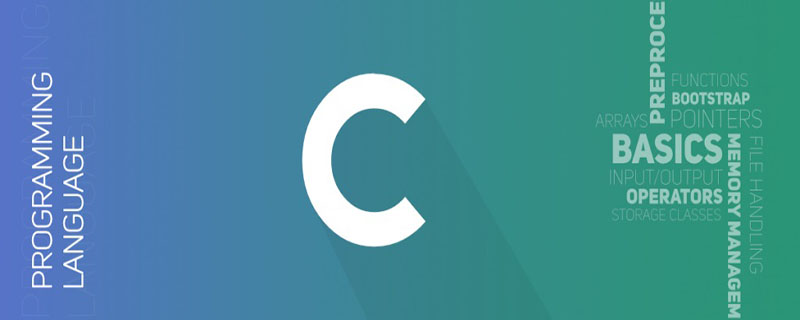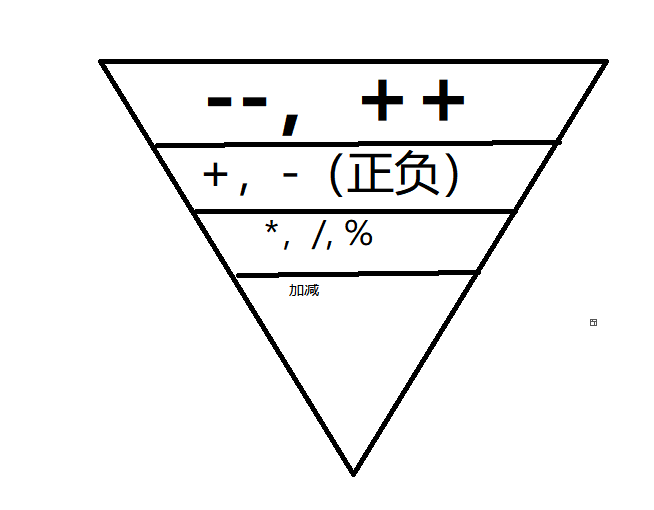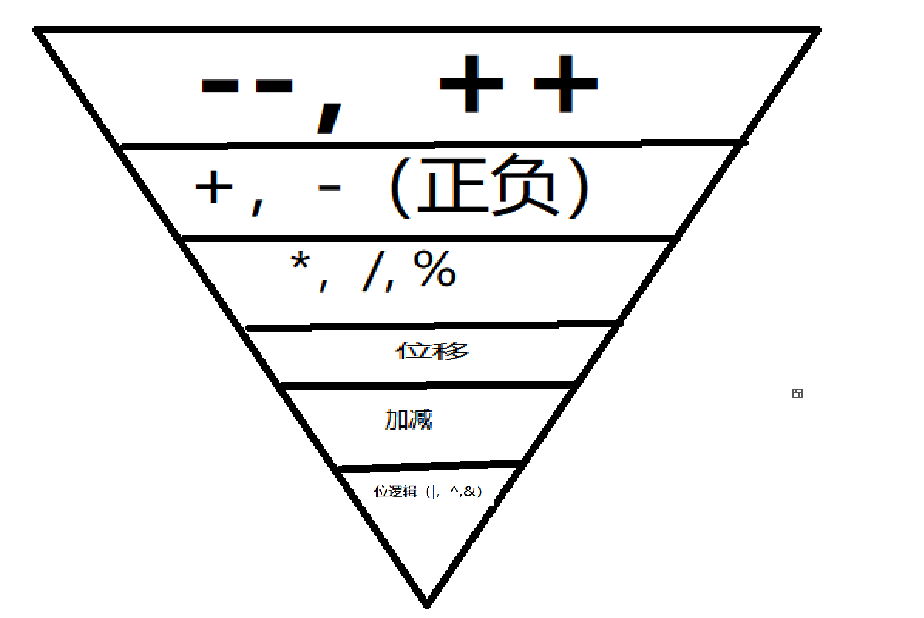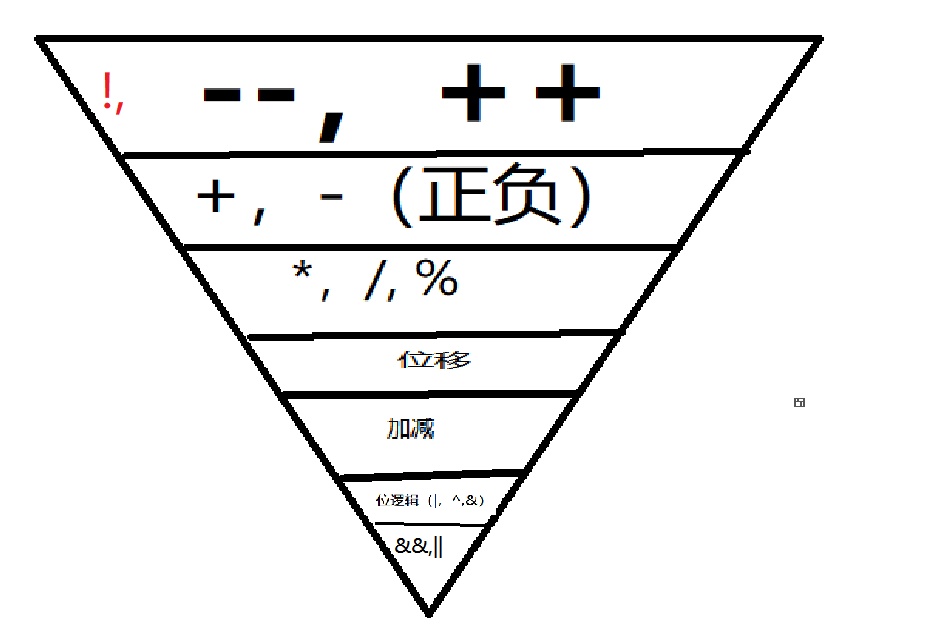• C语言符号优先级
2021-11-06 17:37:57

一共有15级。除了三类结合性是从右到左（单目运算符、三目运算符、=），大多数的运算符都是从左到右。

优先级：算术运算符>关系运算符>逻辑运算符>赋值运算符。逻辑运算符中逻辑非除外。逗号运算符优先级最低

1. []、()、.、->
2. -、（类型）、++、–、*、&、!、~、sizeof
3. /、*、%
4. +、-
5. <<、>>
6. 、>=、<、<=

7. ==、!=
8. &
9. ^
10. |
11. &&
12. ||
13. ?:
14. =、/=、*=、%=、+=、-=、<<=、>>=、&=、^=、|=
15. ,开发语言 后端
更多相关内容
• 1、C语言一共有15个优先级 2、有三种结合方向是从右向左（单目运算符、条件表达式、赋值运算符） 3、同一优先级的运算符，运算次序由结合方向所决定 记忆口诀： 括号成员排第1， 单目运算排第2， 乘除余3、加...

Tips

1、C语言一共有15个优先级

2、有三种结合方向是从右向左（单目运算符、条件表达式、赋值运算符）

3、同一优先级的运算符，运算次序由结合方向所决定

记忆口诀：

括号成员排第1，

单目运算排第2，

乘除余3、加减4，

左移右移排第5，

大于小于6，等于不等于7，

8910：按位与、按位异或、按位或

11和12：逻辑与和逻辑或

13：条件表达式三目运算独一个

各种赋值排14，最后15只剩逗号运算符独一个。

 优先级 运算符 名称或含义 使用形式 结合方向 说明 1 [] 数组下标 数组名[常量表达式] 从左到右 () 圆括号 （表达式）/函数名(形参表) . 成员选择（对象） 对象.成员名 -> 成员选择（指针） 对象指针->成员名 2 - 负号运算符 -表达式 从右到左 单目 运算符 ++ 自增运算符 ++变量名/变量名++ -- 自减运算符 --变量名/变量名-- * 取值运算符 *指针变量 & 取地址运算符 &变量名 ! 逻辑非运算符 !表达式 ~ 按位取反运算符 ~表达式 (类型) 强制类型转换 (数据类型)表达式 sizeof 长度运算符 sizeof(表达式) 3 * 乘 表达式*表达式 从左到右 双目 运算符 / 除 表达式/表达式 % 余数（取模） 整型表达式/整型表达式 4 + 加 表达式+表达式 从左到右 双目 运算符 - 减 表达式-表达式 5 << 左移 变量<<表达式 从左到右 双目 运算符 >> 右移 变量>>表达式 6 > 大于 表达式>表达式 从左到右 双目 运算符 >= 大于等于 表达式>=表达式 < 小于 表达式<表达式 <= 小于等于 表达式<=表达式 7 == 等于 表达式==表达式 从左到右 双目 运算符 != 不等于 表达式!= 表达式 8 & 按位与 表达式&表达式 从左到右 双目 运算符 9 ^ 按位异或 表达式^表达式 从左到右 双目 运算符 10 | 按位或 表达式|表达式 从左到右 双目 运算符 11 && 逻辑与 表达式&&表达式 从左到右 双目 运算符 12 || 逻辑或 表达式||表达式 从左到右 双目 运算符 13 ?: 条件运算符 表达式1? 表达式2: 表达式3 从右到左 三目 运算符 14 = 赋值运算符 变量=表达式 从右到左 += 加后赋值 变量+=表达式 -= 减后赋值 变量-=表达式 /= 除后赋值 变量/=表达式 *= 乘后赋值 变量*=表达式 %= 取模后赋值 变量%=表达式 <<= 左移后赋值 变量<<=表达式 >>= 右移后赋值 变量>>=表达式 &= 按位与后赋值 变量&=表达式 ^= 按位异或后赋值 变量^=表达式 |= 按位或后赋值 变量|=表达式 15 ， 逗号运算符 表达式,表达式,… 从左到右

展开全文开发语言 后端
• C语言中的运算符优先级：1、一级运算符有标识，常量；2、二级运算符有数组下标运算、函数调用；3、三级运算符有前缀自增、前缀自减；4、四级运算符为强制表达式成为指定的类型；5、五级运算符为乘法运算符等等。例如...

C语言中的运算符优先级：1、一级运算符有标识，常量；2、二级运算符有数组下标运算、函数调用；3、三级运算符有前缀自增、前缀自减；4、四级运算符为强制表达式成为指定的类型；5、五级运算符为乘法运算符等等。例如，x = 7 + 3 * 2;在这里，x被赋值为13，而不是20，因为运算符*的优先级高于+，所以它首先与3*2相乘，然后与7相加。

在这里，优先级最高的运算符出现在表的顶部，优先级最低的运算符符出现在表的底部。在表达式中，优先级较高的运算符将首先计算。一级运算符：标识，常量，字符串文字量，优先级提升表达式最优先执行。

二级运算符：数组下标运算(expression)、函数调用(argument-expression-list)、成员访问(identifier、 -> identifier)、后缀自增(i++)、后缀自减(i--)、 复合初始化(initializer-list)。

三级运算符：前缀自增(++i)、前缀自减(--i)、单目转型表式式(取地址& ，提领 * ， 正号+ ，负号-、 位反~ 逻辑否!)、求类型长度(sizeof unary-expression)。

四级运算符：强制表达式成为type-name指定的类型( type-name ) cast-expression。

五级运算符：“ * ” 乘法运算符。

六级运算符：“ + ”加法运算符。

七级运算符：<< 左移运算符；>> 右移运算符。

八级运算符：、>=关系运算符。

九级运算符：“ == ”等于运算符；“ != ”不等于运算符。

十级运算符：“ & ”按位与运算符。

十一级运算符：“ ∧ ”按位异或运算符。

十二级运算符：“ | ”按位或运算符。

十三级运算符：“&&”逻辑与运算符。

十四级运算符：“ || ”逻辑或运算符。

十五级运算符：? :条件运算符。

C语言中运算符优先级如下(从高到底)：运算符关联性

() [] -> . ++ - -左到右

+ - ! ~ ++ - - (type)* & sizeof右到左

* / %左到右

+ -左到右

<< >>左到右

< <= > >=左到右

== !=左到右

&左到右

^左到右

|左到右

&&左到右

||左到右

?:右到左

= += -= *= /= %=>>= <<= &= ^= |=右到左

,左到右

C中的运算符优先级示例：#include main() {

int a = 20;

int b = 10;

int c = 15;

int d = 5;

int e;

e = (a + b) * c / d; // ( 30 * 15 ) / 5

printf("Value of (a + b) * c / d is : %d\n", e );

e = ((a + b) * c) / d; // (30 * 15 ) / 5

printf("Value of ((a + b) * c) / d is : %d\n" , e );

e = (a + b) * (c / d); // (30) * (15/5)

printf("Value of (a + b) * (c / d) is : %d\n", e );

e = a + (b * c) / d; // 20 + (150/5)

printf("Value of a + (b * c) / d is : %d\n" , e );

return 0;}

输出：Value of (a + b) * c / d is : 90

Value of ((a + b) * c) / d is : 90

Value of (a + b) * (c / d) is : 90

Value of a + (b * c) / d is : 50

相关推荐：《C教程》

本篇文章就是关于C语言中的运算符优先级的介绍，希望对需要的朋友有所帮助！

展开全文• 常见符号测试代码如下： void priority_test1() { int a = 2, b = 2, c; //-- 与正负 c = -++a; cout ; cout ; //正负与乘除 a = 10;... 所遇全部符号优先级 a = !0 + 5 && 2; cout ; a = !0 + 5 ; cout ;

常见符号测试代码如下：

void priority_test1() {
int a = 2, b = 2, c;
//-- 与正负
c = -++a;
cout << c << endl;
cout << endl;
//正负与乘除
a = 10;
c = -++a % 4;
cout << c << endl;
c = -++a * 2;
cout << c << endl;
cout << endl;
//*/%
a = 10, b = 4;
c = a % b * b / 3;
cout << c << endl;

//+-与赋值
a = 10, b = 4, c = 2;
c -= a - b;
cout << c << endl;

a = 10, b = 4, c = 2;
c *= (-++a % c * c - b / 2) * 5;
cout << c << endl;
}


结论偏僻符号测试如下

	//位或（逻辑）小于乘除 位移>|
int a = 8, b = 2, c;
c = a << 4 % 3;
cout << c << endl;
cout << endl;

a = 8, b = 2;
c = a << 4 | 0b11;
cout << c << endl;
cout << endl;

a = 8, b = 2;
c = a | 3 << 2;  // 11*4先与
cout << c << endl;
c = a | 12;
cout << c << endl;
cout << endl;

//位移与加减
c = 2 + 1 << 3;
cout << c << endl;

//位移与关系逻辑
c =   1 & 5 << 2;
cout << "c:" << c << endl;
b = 5 << 2;
cout << (2 & b)  << endl;
cout << c << endl;

c =  1 << 2 + 1;
cout << "c:" << c << endl;
c =  1 | 1 << 2 + 1;
cout << "c:" << c << endl;
c =  1 | 1 << 2 | 2;//100==-101--111
cout << "c:" << c << endl;所遇全部符号优先级

	a = !0 + 5 && 2;
cout  << a << endl;
a = !0 + 5 << 2;
cout  << a << endl;展开全文c++
• 今天去翻了下C语言运算符的优先级和结合性，发现当初学习的时候就没认真记住，惭愧。发现一篇讲得不错的文章，编辑了下转来供以后翻阅。   C语言运算符优先级表（由上至下，优先级依次递减）   ...语言 编译器
• 满意答案p3634233推荐于 2017.11.27采纳率：42%等级：9已帮助：4122人下面按优先级从高到低的顺序总结一下各种运算符，每一条所列的各运算符具有相同的优先 级，对于同一优先级的多个运算符按什么顺序计算也有说明，...
• C语言程序设计期末考试试题及其答案一、单项选择题(本大题共20题，每题2分，共...以下不正确的C语言标识符是()A、ABC B、abc C、a_bc D ab.c 3、 一个C语言程序是由() A、一个主程序和若干子程序组成 B、 函数组成 C...
• 1.操作优先级：() [] -> . L-R! ~ ++ -- - (type) * & sizeof R-L* / % L-R+ - L-R>> << L-R< > <= >= L-R== != L-R& L-R^ L-R| L-R&& L-R|| ...
• 运算符优先级 运算符的优先级 初等运算符>单目运算符>算数运算符(先乘除后加减)>移位运算符>关系运算符>位运算符>逻辑运算符>条件运算符>赋值运算符>逗号运算符 初等运算符:圆括号( )...开发语言 后端
• 前端必备的js知识点(转载)1.本文主体源自:http://www.cnblogs.com/coco1s/p/4029708.html,有兴趣的可以直接去那里看,也可以看看我整理加拓展的.2.js是一门什么样的语言及特点? ...移动端rem自适应布局关键... var wW...
• Presentation on theme: "第1讲 C语言基础 要求: (1) C程序的组成 (2) C语言标识符是如何定义的。 (3) C语言有哪些基本数据类型？各种基本数"— Presentation transcript:第1讲 C语言基础 要求: (1) C程序的组成 ...
• 下面按优先级从高到低的顺序总结一下各种运算符，每一条所列的各运算符具有相同的优先 级，...1 、标识符、常量、字符串和用()括号套起来的表达式是组成表达式的最基本单元，在运算中做 操作数，优先级最高。 2、...
• C语言题库(选择题)电子Z1201班 张斌 12109103131.C语言源程序的基本单位是()。A.过程 B.函数 C.子程序 D.标识符 2. 下列字符序列中，可用作C标识符的一组字符序列是()。 A. S.b，sum，average，_above B. class，day...
• 2.2 语句标号 语句标号用标识符表示，它的命名规则与变量名相同，即由字母、数字和下画线组成，在标识符后加一个冒号，就成了一个语句标号。在Ｃ语言中可以在任何语句前加上语句标号。 2.3 goto语句 goto语句为无...
• 1.理解C语言的声明声明主要由声明器、类型说明符、类型限定符和存储属性组成1.1.声明器(declarator)1)指针* const volatile* volatile** const* volatile const指针的五种限定形式2)数组类型 标识符[下标]3)函数类型...
• 希望对初学C语言语言的同志有所帮助； 当然，应用这个口诀的前提是你必须认识所有的C语言的运算符。  优先决 括号成员第一; //括号运算符[]() 成员运算符. -> 全体单目第二; //所有的单目运算符比如++ -...
• 表达式B:表达式C表示对表达式A的值进行判断，如果A为真，则执行表达式B，否则执行表达式C 标识符 C语言里使用名称区分不同内容，诸如变量名，函数名etc这些名称叫做标识符（IdenTIfier），实际开发总是采用驼峰式...
• C语言的单词有如下几种：标识符、关键字、运算符、分隔符、常量、字符串和注释符。下面对上述7种单词的词法规则逐一详述，有些单词，例如，关键字、运算符、常量和字符串等，后面的章节中还会详细讲述。1.标识符...
• ①常量：整型常量、实型常量、字符常量、普通字符、转义字符(表格)、字符串常量、符号常量 ②常变量 ③标识符 \' 单撇号 输出单撇号 ' \" 双撇号 输出双撇号 \? 问号 输出问号字符 \\ 反斜线 输出反斜线...C语言 C语言重要知识点 C语言常量和变量
• 提示：文章写完后，目录可以自动生成，如何生成可参考右边的帮助文档 文章目录 前言 一、pandas是什么？ 二、使用步骤 1.... 2....一、标识符 ...标识符由大小写字母、数字、下划线组成，但不能以..
• C语言程序设计基础习题集一、选择填空知识点1：C程序的基本结构和算法基本概念C语言程序的基本单位为A) 程序行 B) 语句 C) 函数D) 字符 用C语言编写的代码程序A)可立即执行 B)是一个源程序 C)经过编译即可执行 D)...
• 一、选择题1.下述标识符中，()是合法的用户标识符。...在C语言中，字符型数据在内存中是以()形式存放的。A.原码 B.BCD码 C.反码 D.ASCII码3.以下选项中不合法的用户标识符是()。A.abc.c B.file C.Mai...
• C语言中，定义符号常量，变量，数组，结构体，枚举，函数等都需要一个名称，我们把这名称成为标识符。 　2.标识符的命名规则 　2.1标识符只能有字母，数字，下划线，美元（\$）符号组成 　2.2严格区分大小写 　...
• 该楼层疑似违规已被系统折叠隐藏此楼查看此楼各类符号优先级。1 级优先级 左结合() 圆括号[] 下标运算符 指向结构体成员运算符 . 结构体成员运算符2 级优先级 右结合! 逻辑非运算符 ~ 按位取反运算符 ++ 自增运算符 ...
• ·标识符 标识符（identifier）是指用来标识某个实体的一个符号，在不同的应用环境下有不同的含义。在计算机编程语言中，标识符是用户编程时使用的名字，用于给变量、常量、函数、语句块等命名，以建立起名称与使用...
• A.&aB.FORC.pfintD.00 参考答案：A 参考解析：C语言标识符由字母、下划线、数字组成，且开头必须是字母或下划线。另外，关键字不能作为标识符。因为C语言中区分大小写，所以B选项中的。FOR。可以作为标识符来用。...
• ## 【C语言】#define定义的标识符和宏

多人点赞 热门讨论 2022-03-02 13:09:42
C语言】#define定义的标识符和宏、以及宏的优点和缺点、#与##的用法开发语言 后端
• C语言标识符为例，表示标识符的正则表达式如下 (a|b|c…|z|A|B….|Z|_)( (a|b..|z|A|B…|Z|_)|(0|1|2…|9) )* 该表达式看起来很冗长，当引入正则定义后，有: letter_ → a|b|c…|z|A|B….|Z|_ digit → 0|1|2…|9......